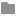# Cubic Elastic constant values for Fe using ADP is exactly half of reported values.

Respected Authority,

Attached Excel sheet contains the comparative results for Ni, Fe Elastic constant (C11cubic, C12cubic, C44cubic) using LAMMPS Elastic example code, for ADP and EAM potentials.

For EAM potential,
The values of Elastic constants are as per the literature OR experiment for both Fe and Ni.

In case of Ni (FCC), Values obtained are also matching with experiment and literature.
But in case of Fe, the values for all three elastic constants are exactly half of the literature values (Table 4, Y.Mishin et. al. |Acta Materialia 53(2005) 4029-4041), . Potential energy value matches exactly with the reported value.

I am not able to figure out the reason, that why the result are deviated for Fe, BCC structure for ADP force field, that too with a factor of 0.5.

If anyone can help in resolving the issue, then it would be very helpful.
Required files and folders are attached.

The results are in output.dat file which are compiled in attached excel sheet.
Here only ADP results are attached.elastic constant

ADP - Miskhin.pdf (278 KB)

Respected Authority,

Attached Excel sheet contains the comparative results for Ni, Fe Elastic constant (C11cubic, C12cubic, C44cubic) using LAMMPS Elastic example code, for ADP and EAM potentials.

For EAM potential,
The values of Elastic constants are as per the literature OR experiment for both Fe and Ni.

In case of Ni (FCC), Values obtained are also matching with experiment and literature.
But in case of Fe, the values for all three elastic constants are exactly half of the literature values (Table 4, Y.Mishin et. al. |Acta Materialia 53(2005) 4029-4041), . Potential energy value matches exactly with the reported value.

I am not able to figure out the reason, that why the result are deviated for Fe, BCC structure for ADP force field, that too with a factor of 0.5.

If anyone can help in resolving the issue, then it would be very helpful.
Required files and folders are attached.

The results are in output.dat file which are compiled in attached excel sheet.
Here only ADP results are attached.elastic constant

ADP - Miskhin.pdf (278 KB)